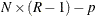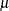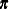The FMM Procedure

Example 39.4 Modeling Multinomial Overdispersion: Town and Country

This example illustrates how you can use the multinomial distribution to model a discrete response that has multiple levels, and how you can use the multinomial cluster model to address overdispersion in multinomial models. The data are survey results from random samples of neighborhoods in both rural and urban areas of Montevideo, Minnesota. There are 18 rural neighborhoods and 17 urban neighborhoods in the survey. In each sampled neighborhood, five households were selected to be interviewed about their level of satisfaction with their homes. The families rated their level of satisfaction as "Unsatisfied," "Satisfied," or "Very Satisfied." These data have previously been analyzed in Brier (1980), Koehler and Wilson (1986), Wilson (1989), and Morel and Nagaraj (1993).

The data include a location type and the numbers of households that respond at each satisfaction level:

data housing;
label us    = 'Unsatisfied'
s     = 'Satisfied'
vs    = 'Very Satisfied';
input type \$ us s vs @@;
datalines;
rural 3 2 0  rural 3 2 0  rural 0 5 0  rural 3 2 0  rural 0 5 0
rural 4 1 0  rural 3 2 0  rural 2 3 0  rural 4 0 1  rural 0 4 1
rural 2 3 0  rural 4 1 0  rural 4 1 0  rural 1 2 2  rural 4 1 0
rural 1 3 1  rural 4 1 0  rural 5 0 0
urban 0 4 1  urban 0 5 0  urban 0 3 2  urban 3 2 0  urban 2 3 0
urban 1 3 1  urban 4 1 0  urban 4 0 1  urban 0 3 2  urban 1 2 2
urban 0 5 0  urban 3 2 0  urban 2 3 0  urban 2 2 1  urban 4 0 1
urban 0 4 1  urban 4 1 0
;


The following DATA step appends two observations that have empty response variables to the data set. These observations are not used in estimating the model parameters, but the FMM procedure scores these observations by using the fitted model.

data toscore;
type='rural'; output;
type='urban'; output;
run;

data housing;
set housing toscore;
run;


The following statements fit a single-component multinomial model to these data, including the location type in the mean model for the multinomial. The response variables are the counts for each observation in vector form.

proc fmm data=housing;
class type;
model us s vs = Type  / dist=multinomial;
output out=Pred pred;
run;


The model includes the only available covariate, Type, as an explanatory variable for the mean of the multinomial distribution. You use the OUTPUT statement and the PRED keyword to direct PROC FMM to include predicted values for each observation in the Pred output data set.

The "Model Information" table in Output 39.4.1 lists the response variables and indicates that this is a single-component multinomial model. The "Fit Statistics" table shows the associated fit statistics for the model.

Output 39.4.1: Model Information and Fit Statistics for the Multinomial Model

The FMM Procedure

Model Information
Data Set WORK.HOUSING
Response Variable us
Response Variable s
Response Variable vs
Type of Model Homogeneous Regression Mixture
Distribution Multinomial
Components 1
Estimation Method Maximum Likelihood

Fit Statistics
-2 Log Likelihood 194.1
AIC (Smaller is Better) 202.1
AICC (Smaller is Better) 203.4
BIC (Smaller is Better) 208.3
Pearson Statistic 107.3

The parameter estimates capture the relationship between the explanatory variable Type and the different response levels, "Unsatisfied," "Satisfied," and "Very Satisfied." To maintain identifiability, the FMM procedure uses two sets of parameters for the three response variables to parameterize this model. Output 39.4.2 shows the resulting parameter estimates.

Output 39.4.2: Parameter Estimates for the Multinomial Model

Parameter Estimates for Multinomial Model
Response Effect type Estimate Standard
Error
z Value Pr > |z|
1 Intercept   0.9163 0.3416 2.68 0.0073
1 type rural 1.3244 0.5813 2.28 0.0227
1 type urban 0 . . .
2 Intercept   1.2763 0.3265 3.91 <.0001
2 type rural 0.7519 0.5770 1.30 0.1925
2 type urban 0 . . .

The Response column indicates the level of the response that is associated with the parameter set. In this model, Response 1 corresponds to the "Unsatisfied" level and Response 2 corresponds to the "Satisfied" level. This corresponds to the order in which you specify the response variables in the MODEL statement. The "Very Satisfied" level does not appear because of identifiability constraints; the corresponding parameter estimates are set to 0, which means that you can treat the "Very Satisfied" level as the reference level. The estimates of the intercept and the rural effect are positive for both of the other levels, indicating that the estimated proportion at the "Very Satisfied" level is smaller than the proportion at the other two levels for both rural and urban locations.

The Pred output data set contains predicted proportions for each location type. The following statements display the observations that have empty responses and their associated predictions:

proc print data=pred(where=(us=.)) noobs;
var type pred:;
run;


Output 39.4.3 shows the predicted proportions at each response level for each location type. As in Output 39.4.2, the order reflects the order in which you specified the responses in the MODEL statement. Pred_1 corresponds to "Unsatisfied", Pred_2 corresponds to "Satisfied," and Pred_3 corresponds to "Very Satisfied."

Output 39.4.3: Predicted Proportions for Multinomial

type Pred_1 Pred_2 Pred_3
rural 0.52222 0.42222 0.05556
urban 0.35294 0.50588 0.14118

The estimates of response proportions for the two location types indicate a difference in the distribution of satisfaction levels for the rural and urban populations. In particular, the urban population shows a smaller proportion of respondents in the "Unsatisfied" category (Pred_1).

The number of degrees of freedom is, where N is the number of observations, R is the number of levels in the multinomial response, and p is the number of parameters in the model. The ratio of the Pearson statistic to the degrees of freedom is then 107.3 / (352 – 4) = 1.625; this is larger than 1 and so indicates potential overdispersion.

One explanation for overdispersion might be correlation. It is likely that the families in these households meet and talk with one another, which might result in some influence of opinions about housing satisfaction. The observations are not independent in this case; if you model the proportion of each level of satisfaction based only on location type, you will miss this interhousehold influence.

The multinomial cluster model (Morel and Nagaraj 1993) is based on the idea of "clumping"; that is, some proportionof the observed population responds in the same way. In the context of the housing satisfaction data, this means that the clumped responders all express the same satisfaction level. The remaining households respond according to a multinomial distribution with parameter.

In this model, the clumped responders respond identically with one of the three levels of satisfaction, and that level is not observable. This discrete latent factor makes a mixture of three multinomials an appropriate method. The difference between this mixture and a general mixture of multinomials is the role of the clumping proportionand the use of the mixing probabilities in the mean model. In this model, the mixing probabilitiesalso define the multinomial distribution that governs the distribution of the non-clumped responses.

The following statements fit a multinomial cluster model to these data:

proc fmm data=housing;
class type;
model us s vs = Type / dist=multinomcluster;
output out=Pred pred;
probmodel Type;
run;


You include Type in the mean for the underlying multinomial distribution by using the PROBMODEL statement and also in the mean for the clumping parameterby using the MODEL statement. Output 39.4.4 shows model information and fit statistics for this multinomial cluster model. Because the model specifies three response variables, the resulting mixture model has three components.

Output 39.4.4: Model Information and Fit Statistics for the Multinomial Cluster Model

The FMM Procedure

Model Information
Data Set WORK.HOUSING
Response Variable us
Response Variable s
Response Variable vs
Type of Model Multinomial Cluster
Distribution Multinomial Cluster
Components 3
Estimation Method Maximum Likelihood

Fit Statistics
-2 Log Likelihood 182.9
AIC (Smaller is Better) 194.9
AICC (Smaller is Better) 197.9
BIC (Smaller is Better) 204.3
Pearson Statistic 61.9809
Effective Parameters 6
Effective Components 3

The fit statistics are generally better for the multinomial cluster model. However, Output 39.4.5 indicates that the parameters in the mean model for the clumping probabilityare not significantly different from 0. There does not appear to be strong evidence for a clumping effect as modeled by the multinomial cluster model.

Output 39.4.5: Parameter Estimates for the Multinomial Cluster Model

Parameter Estimates for Multinomial Cluster Model
Component Effect type Estimate Standard
Error
z Value Pr > |z|
1 Intercept   -0.3696 0.4385 -0.84 0.3992
1 type rural 0.09401 0.6312 0.15 0.8816
1 type urban 0 . . .

In the multinomial cluster model, the predicted proportions are the same as the mixing probabilities. Output 39.4.6 shows the parameter estimates for the mixing probabilities.

Output 39.4.6: Mixing Probability Parameter Estimates for the Multinomial Cluster Model

Parameter Estimates for Mixing Probabilities
Component Effect type Estimate Standard
Error
z Value Pr > |z|
1 Intercept   0.6383 0.4106 1.55 0.1201
1 type rural 1.4138 0.6781 2.08 0.0371
1 type urban 0 . . .
2 Intercept   1.1077 0.3741 2.96 0.0031
2 type rural 0.7900 0.6527 1.21 0.2262
2 type urban 0 . . .

As in the multinomial example, the estimates for the intercept and rural effect are positive for both the "Unsatisfied" and "Satisfied" response levels, indicating that these levels have larger predicted proportions than the "Very Satisfied" level.

Output 39.4.7 shows the predicted proportions at each level of the response for each location type.

Output 39.4.7: Predicted Proportions for the Multinomial Cluster Model

type Pred_1 Pred_2 Pred_3
rural 0.50367 0.43163 0.06471
urban 0.31977 0.51133 0.16890

By comparing Output 39.4.7 with Output 39.4.3, you can see that the proportion estimates are not markedly different between the models. This is consistent with the lack of significance in the multinomial cluster model’s clumping parameters.